# Math Brain Teasers 7:: Solve The Triangle Puzzle - What Number Should Replace Question Mark?

in STEMGeeks2 years ago

Hey All;

Today, I've for you all a Simple Triangle Puzzle to be solved. There is no denying the fact that solving Math problems or puzzles require logic. And in order to solve this Triangle Puzzle you will need to find the logic and then only will you be able to solve this puzzle.

Have a close look at both Triangles which are solved for you. Find the Number which should replace the Question Mark?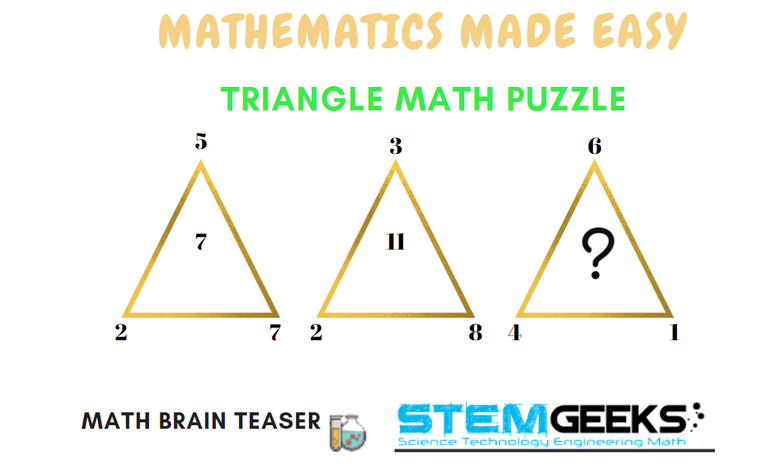I don't want to scare you by saying that you need to find the logic. The logic isn't that complex and just involves simple calculations. Let me give you a hint to solve this triangle picture puzzle.

Hint:: Here is a simple hint to get you started, solving this puzzle. The numbers that are written on the Triangle edges have to be moved in an Anti-Clock direction. This is BIG; Hint that I've given you ALL. Let's see now if you are able to solve this Triangle Math Puzzle.

### Math Brain Teasers 6:: Solve the Picture Puzzle? - Solved with Explanation

Let's have a look at the answers and the math/logic involved in finding to:: Solve the Picture Puzzle? for our SIXTH Math Brain Teasers 6 Question.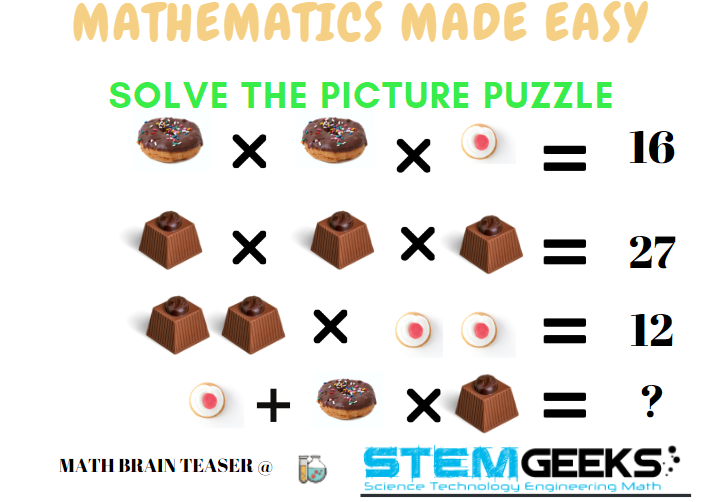### Logic to Finding; Solve the Picture Puzzle?

• Step 1:: In order to solve this puzzle, Let us use the following notations::

• Donut = D

• Cupcake = C

• Chocolate = L

• Step 2 :: By looking at the second equation it will give us::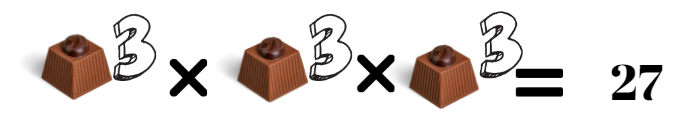### The Value of One Chocolate i.e. L = 3

• Step 3:: Let us now try to solve the third equation now::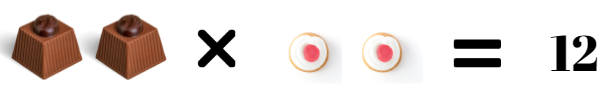L = 3 ; found in Step 1

L L * 2 C = 12

6 *2 C = 12/6

C = 2/2 = 1

### Hence 1 Cupcake i.e. C = 1

• Step 4 :: Lets now try to solve the 1st equation to find the value of Donut

C = 1; from Step 3

D * D * C = 16

4 * 4 * 1 = 16

### Hence Donut i.e. D = 4

Finally, solve the equation in question with the following::

The Value of One Chocolate i.e. L = 3
1 Cupcake i.e. C = 1
Hence Donut i.e. D = 4

Let's see the equation in Question now::

C + D X L = ?

1 + 4 x 3 = 1 + 12 => 13

Hence the answer to the Math Brain Teasers 6:: Solve the Picture Puzzle? = 13

### STEM token GiveAway

I'll be again doing a giveaway of STEM tokens to the lucky random winner with the correct answer. We had 9 entries & out of which 5 entries were correct. And that is of @acont;@dobro2020 ; @dkmathstats; @saachi; & @pimpstudio-cash.. And the WINNER is::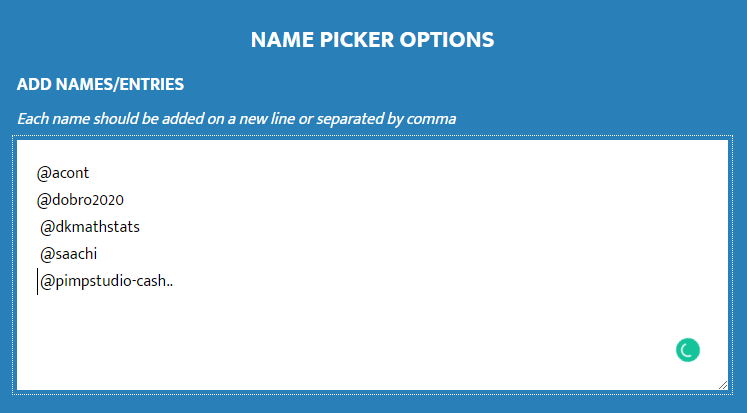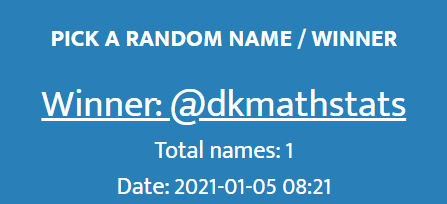Congratulations @dkmathstats; Your WIN yourself 10 STEM tokens; worth 2.2+ HIVE. You should be having the rewards in your STEM Wallet Soon.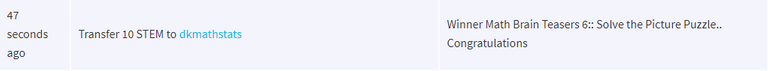### Math Quote for the Day::

Here is the motivation to solve this Math Brain Teasers 7:: Solve The Triangle Puzzle?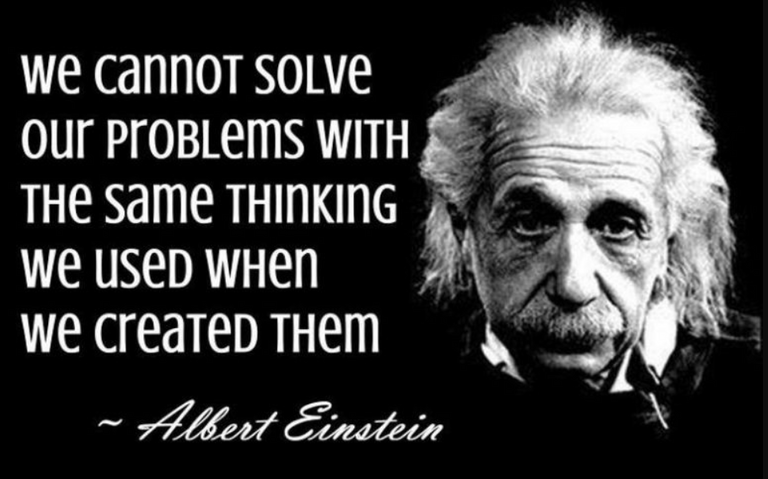source::

If you like my work, then please spread the Word.. that we do have the Math Brain Teasers competition here @StemGeeks platform. Reblog is much appreciated.

Best Regards

PS:: All the Maths Brain Teasers; are made by me using the Pro Canva License Version

Posted with STEMGeeks

Sort:
2 years ago (edited)

-8 [D = 2xC - (A+B)]

Ahh way above my head . Did multiple tries but not able to arrive at common answer but will be waiting to see what the answer is tomorrow or by others.

7

I guess 5.

-6

2 years ago (edited)

5

Posted using Dapplr

My answer is 7 but not really very sure.

I guess 13 but not so sure. That was a great brain teaser

I am guessing 10

!wine

Cheers, @asimo You Successfully Shared 0.100 WINE With @gungunkrishu.
You Earned 0.100 WINE As Curation Reward.
You Utilized 2/3 Successful Calls.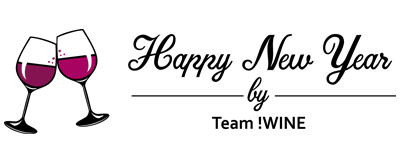WINE Current Market Price : 0.000 HIVE